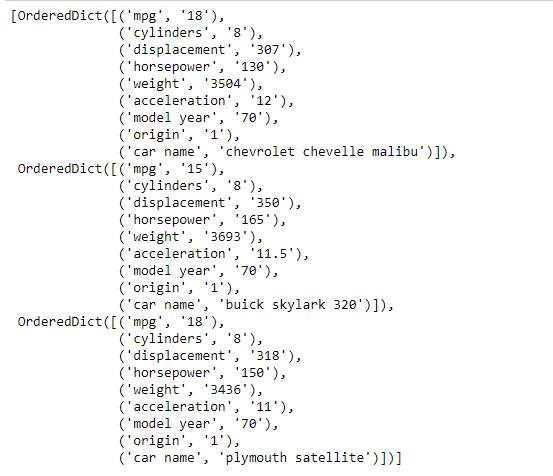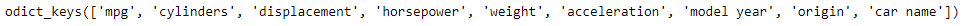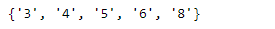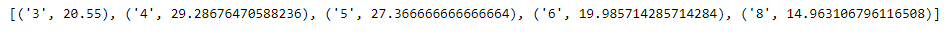# Using csv module to read the data in Pandas

• Last Updated : 05 Aug, 2021

The so-called CSV (Comma Separated Values) format is the most common import and export format for spreadsheets and databases. There were various formats of CSV until its standardization. The lack of a well-defined standard means that subtle differences often exist in the data produced and consumed by different applications. These differences can make it annoying to process CSV files from multiple sources. For that purpose, we will use Python’s csv library to read and write tabular data in CSV format.
Code #1: We will use csv.DictReader() function to import the data file into the Python’s environment.

## Python3

 `# importing the csv module``import` `csv` `# Now let's read the file named 'auto-mpg.csv'``# After reading as a dictionary convert``# it to Python's list``with ``open``(``'auto-mpg.csv'``) as csvfile:``    ``mpg_data ``=` `list``(csv.DictReader(csvfile))` `# Let's visualize the data``# We are printing only first three elements``print``(mpg_data[:``3``])`

Output :As we can see the data is stored as a list of ordered dictionary. Let’s perform some operations on the data for better understanding.
Code #2:

## Python3

 `# Let's find all the keys in the dictionary``print``(mpg_data[``0``].keys)` `# Now we would like to find out the number of``# unique values of cylinders in the car in our dataset``# We create a set containing the cylinders value``unique_cyl ``=` `set``(data[``'cylinders'``] ``for` `data ``in` `mpg_data)` `# Let's print the values``print``(unique_cyl)`

Output :As we can see in the output, we have 5 unique values of cylinders in our dataset.
Code #3: Now let’s find out the value of average mpg for each value of cylinders.

## Python3

 `# Let's create an empty list to store the values``# of average mpg for each cylinder``avg_mpg ``=` `[]` `# c is the current cylinder size``for` `c ``in` `unique_cyl:``    ``# for storing the sum of mpg``    ``mpgbycyl ``=` `0``    ``# for storing the sum of cylinder``    ``# in each category``    ``cylcount ``=` `0` `    ``# iterate over all the data in mpg``    ``for` `x ``in` `mpg_data:``        ``# Check if current value matches c``        ``if` `x[``'cylinders'``]``=``=` `c:``            ``# Add the mpg values for c``            ``mpgbycyl ``+``=` `float``(x[``'mpg'``])``            ``# increment the count of cylinder``            ``cylcount ``+``=` `1` `    ``# Find the average mpg for size c``    ``avg ``=` `mpgbycyl``/``cylcount``    ``# Append the average mpg to list``    ``avg_mpg.append((c, avg))` `# Sort the list``avg_mpg.sort(key ``=` `lambda` `x : x[``0``])` `# Print the list``print``(avg_mpg)`

Output :As we can see in the output, the program has successfully returned a list of tuples containing the average mpg for each unique cylinder type in our dataset.

My Personal Notes arrow_drop_up